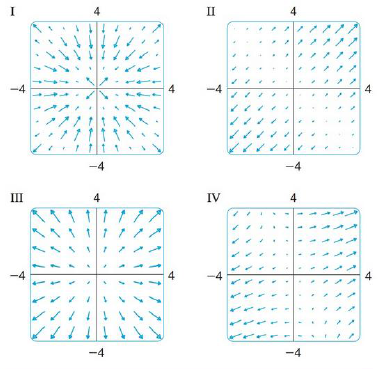Chapter 16.1, Problem 32E

Chapter
Section
Textbook Problem

Match the functions f with the plots of their gradient vector fields labeled I-IV. Give reasons for your choices.32. f(x, y) = sin x 2 + y 2To determine

To match: The function f(x,y)=sinx2+y2 with the plots labeled as I-IV.

Explanation

Given data:

f(x,y)=sinx2+y2

Formula used:

Write the expression for gradient vector field of two dimensional vector.

f(x,y)=fxi+fyj (1)

Write the required differentiation formulae with respect to x as follows.

x(sinx)=cosxx(x)=12xx(x2)=2xx(y2)=0

Write the required differentiation formulae with respect to y as follows.

y(siny)=cosyy(y)=12yy(y2)=2yy(x2)=0

Differentiate the term sinx2+y2 with respect to x .

x(sinx2+y2)=(cosx2+y2)xx2+y2=(cosx2+y2)12x2+y2x(x2+y2)=(cosx2+y2)12x2+y2(2x+0)=xcosx2+y2x2+y2

Differentiate the term sinx2+y2 with respect to y

Still sussing out bartleby?

Check out a sample textbook solution.

See a sample solution

The Solution to Your Study Problems

Bartleby provides explanations to thousands of textbook problems written by our experts, many with advanced degrees!

Get Started

Let x be the function defined by s(t)=2tt21. Find s(4), s(0), s(a). s(2 + a), and s(t + 1).

Applied Calculus for the Managerial, Life, and Social Sciences: A Brief Approach

Reduce to lowest terms. 9.

Contemporary Mathematics for Business & Consumers

In Problems 1-6, graph each inequality. 2.

Mathematical Applications for the Management, Life, and Social Sciences

Evaluate the integral, if it exists. sinxcos(cosx)dx

Single Variable Calculus: Early Transcendentals

For y = x sin x, y = _____. a) x cos x b) x cos x + 1 c) cos x d) x cos x + sin x

Study Guide for Stewart's Single Variable Calculus: Early Transcendentals, 8th

True or False: converges conditionally.

Study Guide for Stewart's Multivariable Calculus, 8th

In Exercises 918, indicate whether the matrix is in row-reduced form. [1100|30]

Finite Mathematics for the Managerial, Life, and Social Sciences

Define the concept of external validity and a threat to external validity.

Research Methods for the Behavioral Sciences (MindTap Course List)